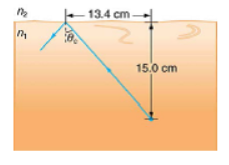# Problem: A ray of light, emitted beneath the surface of an unknown liquid with air above it, undergoes total internal reflection as shown in Figure 25.54. What is the index of refraction for the liquid and its likely identification?Figure 25.54 A light ray inside strikes the surface at the critical angle and undergoes total internal reflection.

###### FREE Expert Solution

With a critical angle, Snell's law:

$\overline{)\begin{array}{rcl}{\mathbf{n}}_{\mathbf{1}}\mathbf{s}\mathbf{i}\mathbf{n}{\mathbf{\theta }}_{\mathbf{c}}& {\mathbf{=}}& {\mathbf{n}}_{\mathbf{2}}\\ {\mathbf{n}}_{\mathbf{1}}& {\mathbf{=}}& \frac{{\mathbf{n}}_{\mathbf{2}}}{\mathbf{s}\mathbf{i}\mathbf{n}{\mathbf{\theta }}_{\mathbf{c}}}\end{array}}$

Critical angle, θc:

$\overline{){{\mathbf{\theta }}}_{{\mathbf{c}}}{\mathbf{=}}{\mathbf{s}}{\mathbf{i}}{{\mathbf{n}}}^{\mathbf{-}\mathbf{1}}{\mathbf{\left(}}\frac{{\mathbf{n}}_{\mathbf{2}}}{{\mathbf{n}}_{\mathbf{1}}}{\mathbf{\right)}}}$

99% (442 ratings)###### Problem Details

A ray of light, emitted beneath the surface of an unknown liquid with air above it, undergoes total internal reflection as shown in Figure 25.54. What is the index of refraction for the liquid and its likely identification?Figure 25.54 A light ray inside strikes the surface at the critical angle and undergoes total internal reflection.